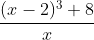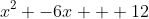## Friday, August 13, 2010

### Calculus Questionnaire

I gave a questionnaire to my calculus courses (one section of honors calculus, one of AP calculus AB, one of AP calculus BC) to get their minds going and preview some of the things we're doing. Below are the questions and why I asked them as well as any interesting answers given.

1. What is the furthest you've been away from home?
• Why I asked: On a personal level, I wanted to know where some of my students have been. On a mathematical level, I wanted them to think about practical distances and how we measure distance. (Nobody said it, but I mentioned things like, "If you step out your front door, that might be the furthest away if you measure around the earth the other way. But why stop at measuring around the earth? Why not measure around the sun?")

2.What is the largest number you can write in the space below?
• Why I asked: Getting at the concept of infinity, but I wanted to point out that infinity is not a number (at least not in the calculus we're doing). Creative answers would've also been good like a large-font 8 or something.
• Interesting answers: ZERO (written in tall letters), "a really big number," 9^9^9^9^9, infinity, "I'm not playing this game"

3. What is the least probable (but still possible) event that you can think of? If you had to give it a percent chance of happening, what would it be?
• Why I asked: Getting at the concept of limits, specifically as x approaches 0 from the right. Also interesting to see creativity and interests.
• Interesting answers: scoring an 18 on a professional golf course, being slapped by a walrus, gingers ever being cool, a squirrel causing a 30 car pileup, someone dying because they wore a black vest and choked on the buttons (what?), failing this class (yeah!), meeting everyone in the world, everyone getting along (:(), walking through a wall because your atoms pass through the spaces and reconfiguring on the other side

4. What is the number right before 4?
• Why I asked: Another attempt to get at limits. Also pointing out that 3.9999...(repeating) is not the answer because it actually is 4 (we'll learn later in the series section). I expected a lot of them to put 3 or 3.9(repeating). Maybe "4" because on the paper it's the previous number before the "4" from the end of the question.

5. Have you taken or are you taking physics?
• Why I asked: It's not necessary for calculus, but our school doesn't offer calculus-based physics. So, those who have taken or are taking it can see some cool stuff like where the kinematics equations come from.

6. Do you know the difference between the meaning of the words "velocity" and "speed?" What about "displacement" and "distance traveled?"
• Why I asked: Straightforward. This may not be a necessary question in the future, but it gave me an opportunity to tell them one of the basic applications of calculus.

7. Using algebra skills, can you turn this:into this:• Why I asked: Practicing algebra using the difference quotient. We'll be coming up on these types of questions in the next week or so and I wanted to get a sense of where they were and give them an idea of what level of math we're looking at.

8. Using precalculus knowledge, write an equivalent expression for each of the following using trig identities you learned:

9. In as many steps as possible, explain how to make a bowl of cereal.
• Why I asked: I thought it would be interesting mathematically to talk about how many steps we have to show at this level (eg If you have a quadratic, can you just write the answer on the next line or do you have to show the quadratic formula and all that?). I also wanted to talk about how mathematicians think in details. How far back would you go? (Go to the store to buy cereal? Grow wheat to make the cereal? Evolve plants to make the wheat? etc.) And what level of detail would you go into? (Send a signal from your brain to your arm muscle to extend your arm towards the spoon.)
• Interesting answers: Really, there are too many to list here, but one kid wrote more than 80 steps. Another had some sort of story in his where you go to the store to choose your cereal and then a few steps about trying pickup lines on the checkout girl.

10. Find the shaded area of the shape in the picture below (Picture of a polygon that is a rectangle with triangles sticking out of each side.)
• Why I asked: I wanted them to get started on thinking about integral calculus where we take a region and find its area by dividing it into smaller regions. Also, in broader terms, just taking a large problem and breaking it down into smaller, more manageable parts (see Sam's motto "turn what you don't know into what you do know").

11. Find the following:
• The slope of a line passing through the points (-1,2) and (3,-1).
• An equation of the line that goes through the points (2,4) and (5,2).
• An equation of a line with slope 0.
• Something in the school that has a positive slope

• Why I asked: Basic review. I also pointed out that the last part can be tricky, because if you go to the other side of the "up staircase," then it's a "down staircase" and has negative slope.
• Interesting answers: Knowledge gained, age of a student

12. What do you think calculus is?
• Why I asked: I thought it'd be interesting to know how many students actually knew what they were getting into. (Very few did.)
• Interesting answers: A difficult class that can only be understood when taught, the next class after precalculus, something to do with curves, I have no idea, hard math, the highest math class

13. Write the word "minimum" below in cursive.
• Why I asked: I always thought it was a fun word to write. You kinda scribble up and down a lot and then go back and dot some i's.

14. Haha! Wasn't that fun? Now, why are you taking this class?
• Why I asked: I thought it would be interesting to read the different reasons they thought of--especially in light of #12 on this questionnaire.
• Interesting answers: because it looks good on college apps, because it's next after precal, I wanted to take a math my senior year and I don't like statistics, I like punishing myself with challenging math classes, good question

Overall, I think it was a fun experience for them. It was interesting to note the difference in answers from the "creative" people from the "beaten-down-by-the-system" people. A couple kids in my BC class said, "You're going to hate me when you read my answers, Mr. P." When I asked why, they'd say, "Well, like for the 'biggest number' question, I just wrote a huge number 2." I tried not to smile too big.

1.I like the questions! There's a nice shortcut for #7 if students remember the sum/difference of cubes formulas.

How much time was given for this?

2.I gave it to them on the way out the first day and took it up the day after, so it was more of a homework sort of thing. We went over the answers, though, before I took it up and gave them an opportunity to share some of their more creative answers in class. I don't think they took advantage of the binomial theorem/pascal's triangle for expanding the cubic in #7 and they definitely didn't think of factoring it. So, they did the ol' (x-2)(x-2)(x-2) "FOIL" thing (those who did get it).

3.If 4 is the number before 4, haven't all of your students already taken calculus?

Cool questionnaire.

4.Thanks, I've modified it and will use with my college class.

5.Thanks! I used this on the first day and loved their answers to #3. I also noticed they were pretty vague on "What is Calculus", which led nicely into the first lesson on pre-calculus vs calculus mathematics.